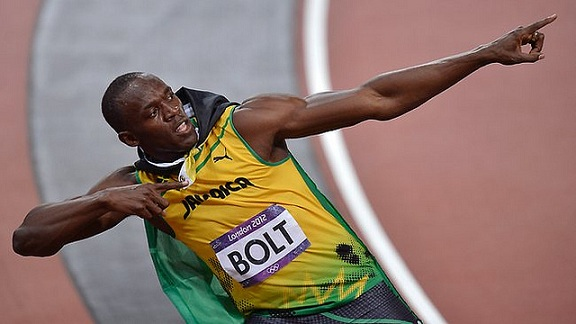# Difference Between Acceleration and Deceleration

## Main Difference – Acceleration vs. Deceleration

Acceleration and deceleration are two of the most basic concepts encountered in mechanics. The main difference between acceleration and deceleration is that acceleration refers to a rate of change of velocity while deceleration refers to a value of acceleration which is negative.

## What is Acceleration

In physics, when a body changes its position, we quantify the change in position in terms of a quantity called displacement. Displacement is a vector quantity. Its size is the distance between the body’s starting position and the final position. The direction of the displacement vector is in the direction pointing from the starting position to the final position. The rate of change of displacement gives the object’s velocity. If the displacement is given by$\vec{r}$, then the velocity$\vec{v}$ is:$\vec{v}=\frac{\mathrm d\vec{r}}{\mathrm dt}$

Acceleration is the rate of change of velocity. If acceleration is given by$\vec{a}$, then it is defined:$\vec{a}=\frac{\mathrm d\vec{v}}{\mathrm dt}=\frac{\mathrm d^2\vec{r}}{\mathrm dt^2}$

Note that since velocity is a vector quantity, acceleration could mean a change in either the speed or direction of an object. An object travelling in a curved path at constant speed is still accelerating!

In the mathematical sense, an acceleration could either increase or decrease an object’s speed. In everyday usage, however, when we talk about accelerations, we are talking about things speeding up rather than slowing down. Sprinters, especially those sprinting for short distances, need to increase their speeds at a very short time. For instance, when Usain Bolt set the world record for the men’s 100 m sprint, he reached an acceleration of about 9.5 m s-2 at the start.In his record-breaking 100 m sprint, Usain Bolt accelerated at about 9.5 m s-2.

## What is Deceleration

Since acceleration is a vector, it could take either positive or negative values. “Deceleration” is a term given to a negative acceleration. This means that the speed is decreasing over time. According to Newton’s second law, if there is an acceleration there is an unbalanced force involved. If the resultant force is acting on an object in the direction of the object’s motion, the object would have a positive acceleration: i.e. its speed would increase. If the resultant force is acting on an object in the direction against the object’s direction of motion, then the object would have a negative acceleration: i.e. its speed would decrease and the object would eventually come to a stop. If the resultant force continues to act, the object would proceed to speed up in the direction opposite to the direction in which it was initially travelling.

## Difference Between Acceleration and Deceleration

### Definition

Acceleration is the rate of change of velocity.

Deceleration is a negative acceleration.

### Direction of Forces

Acceleration happens when there is a resultant force on an object, in any direction.

Deceleration takes place when a resultant force acts on an object in the direction opposite to  the direction in which it is moving.

### Non-technical Usage

In everyday terms, an acceleration refers to an increase in speed.

In everyday terms, a deceleration refers to a reduction in speed.

References

Dvorsky, G. (2013, July 26). The Physics of Usain Bolt’s World Record 100-meter Dash. Retrieved December 1, 2015, from io9 – We come from the future.

Image Courtesy

“599806-100m-final-usain-bolt” by drcliffordchoi (CC BY 2.0) [CC BY-SA 2.0], via flickr